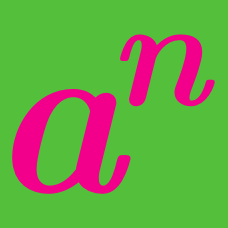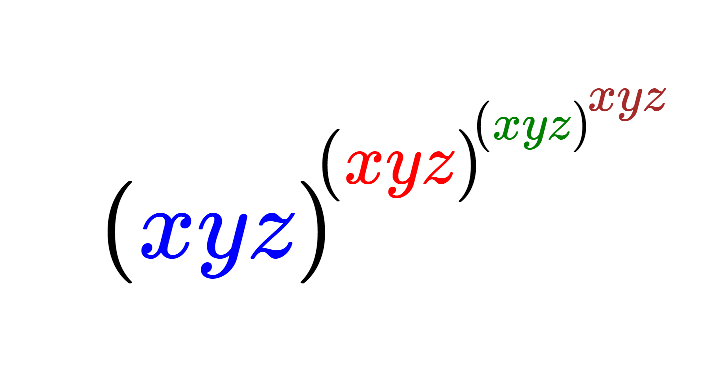Algebra

# Exponential Functions: Level 2 Challenges

$\large 2^x=\dfrac{1}{2^y}, \quad\quad\quad x + y = \ ?$

$\large 2^{x} = 3^{y} = 12^{z}$

If the equation above is fulfilled for non-zero values of $$x,y,z,$$ find the value of $$\frac { z(x+2y) }{ xy }$$.

$\large{ \begin{cases} ab = a^b \\\\ \frac{a}{b} = a ^ {3b} \\ \end{cases}}$

$$a$$ and $$b$$ are real numbers such that $$a > 1$$ and $$b \neq 0$$, satisfying the above system.

Find $$b ^ { -a}.$$

$\large 5^{2x}+5^5=5^{x+3}+5^{x+2}$

Find the sum of the solutions of $$x$$ that satisfy the equation above.Given that $$x,y,z$$ are positive real numbers that satisfy the equations: $$x = y^z, y=z^x, z = x^y$$, find the value of the expression above.

×